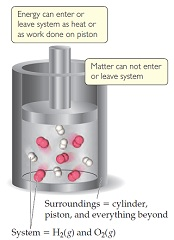# Problem: A gas is confined to a cylinder under constant atmospheric pressure, as illustrated in the following figure. When the gas undergoes a particular chemical reaction, it absorbs 826 J of heat from its surroundings and has 0.66 kJ of P-V work done on it by its surroundings.What is the value of E for this process?

###### FREE Expert Solution

We are being asked to calculate ΔE or the internal energy of the system.

The internal energy (ΔE or ΔU) of a system can be calculated from the heat and work of the system. The relationship between internal energy, heat, and work is shown in the following equation:

ΔE = internal energy, J
q = heat, J
w = work, J###### Problem Details
A gas is confined to a cylinder under constant atmospheric pressure, as illustrated in the following figure. When the gas undergoes a particular chemical reaction, it absorbs 826 J of heat from its surroundings and has 0.66 kJ of P-V work done on it by its surroundings.What is the value of E for this process?